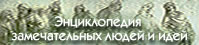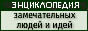Golden Mean Golden Mean, Fibonacci series, Golden rectangles, phi=1.6180339887499
The Golden Mean
Fibonacci series, Golden rectangles, phi=1.6180339887499

The Golden Mean (or Golden Section), represented by the Greek letter phi, is one of those mysterious natural numbers, like e or pi, that seem to arise out of the basic structure of our cosmos. Unlike those abstract numbers, however, phi appears clearly and regularly in the realm of things that grow and unfold in steps, and that includes living things.
The decimal representation of phi is 1.6180339887499...

Number Series
If you start with the numbers 0 and 1, and make a list in which each new number is the sum of the previous two, you get a list like this:
0, 1, 1, 2, 3, 5, 8, 13, 21, 34, 55, 89, 144, ... to infinity-->

This is called a 'Fibonacci series'.
If you then take the ratio of any two sequential numbers in this series, you'll find that it falls into an increasingly narrow range:

1/0 = Whoa! That one doesn't count.
1/1 = 1
2/1 = 2
3/2 = 1.5
5/3 = 1.6666...
8/5 = 1.6
13/8 = 1.625
21/13 = 1.61538...
34/21 = 1.61904...
and so on, with each addition coming ever closer to multiplying by some as-yet-undetermined number.

The number that this ratio is oscillating around is phi (1.6180339887499...). It's interesting to note that the ratio 21/13 differs from phi by less than .003, and 34/21 by only about .001 (less than 1/10 of one percent!), thus providing our less technically-advanced ancestors an easy way to derive phi on a large scale in the real world with a high degree of precision.

Geometry
If you have a rectangle whose sides are related by phi (say, for instance, 13 x 8), that rectangle is said to be a Golden Rectangle. It has the interesting property that, if you create a new rectangle by 'swinging' the long side around one of its ends to create a new long side, then that new rectangle is also Golden. In the case of our 13 x 8 rectangle, the new rectangle will be (13 + 8 =) 21 x 13. You can see this is the same thing that's going on in our number list, but when you discover it geometrically it looks downright magical. If you start with a square (1 x 1) and start swinging sides to make rectangles, you wind up with Golden rectangles without even trying. Here's the list, in case it isn't obvious:
1 x 1
2 x 1
3 x 2
5 x 3
8 x 5
13 x 8
21 x 13
34 x 21
and so on, with, again, each addition coming ever closer to multiplying by phi.
Ancient architecture is filled with Golden rectangles.
Unfold the Golden Rectangle
Text-only version (character-based graphics)
When you swing the long side of a Golden Rectangle around to create a new rectangle, the line it forms with the short side is made up of two sections having lengths of phi and one, respectively. This division of a straight line into a phi proportion is what is actually meant by the term 'Golden Section'.

Pure Math
Proportion is the relationship of the size of two things.
Arithmetic proportion exists when a quantity is changed by adding some amount.
Geometric proportion exists when a quantity is changed by multiplying by some amount.
Phi possesses both qualities.
If you study the Fibonacci series and the Golden Rectangle carefully, you will eventually realize that
phi + 1 = phi * phi.
Consider: suppose that you start with a Golden rectangle having a short side one unit long. Since the long side of a Golden rectangle equals the short side multiplied by phi, the long side of our rectangle is one times phi. or simply phi.
Now suppose that you swing the long side to make a new Golden rectangle. The short side of the new rectangle is, of course, phi units long, and the long side is that times phi, or phi * phi. This describes a Geometric proportion.
But we also know from simple geometry that the new long side equals the sum of the two sides of the original rectangle, or phi + 1. This describes an Arithmetic proportion.
Since these two expressions describe the same thing, they are equivalent, and so
phi + 1 = phi * phi.
(Now that we know this, we can discover the exact value of phi. )
The resulting proportion is both arithmetic and geometric. It is thus perfect proportion; you could think of it as the place on some imaginary graph where the curved line of multiplication crosses the straight line of addition.
Derive phi graphically See the 'imaginary' graph.

Nature
In pure mathematics, an increase in size can be any imaginable number, even one like e or pi. But in the world of nature, things always grow by adding some unit, even if the unit is as small as a molecule. So it's not surprising that phi turns out to be an ideal rate of growth for things which grow by adding some quantity.
Some examples:
The Nautilus shell (Nautilus pompilius) grows larger on each spiral by phi.
The sunflower has 55 (see number list) clockwise spirals overlaid on either 34 or 89 (see number list) counterclockwise spirals, a phi proportion.

Back to GOLDEN PROPORTIONCopyright © 2004 abc-people.com Design and conception BeStudio © 2004-2013